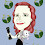## Friday, September 24, 2010

### Charts of different pch values in R

I always forget what each pch values is and I always end up going to this site to see the list: http://rgraphics.limnology.wisc.edu/pch.php. But I don't like having to click on the thumbnail image to then see the large version. Here is my version:
I don't understand why it has the value '0', and if there is a difference between 16 and 19 I have no idea what it is. and of course 20 could just be 19 with cex=.5

Here are some other pch options, basically any character on the keyboard can be used:
Of course some of these are better than others. For example, if you are plotting financial data, it might be best to consider something like this:

Or if you have confusing data this may be appropriate:

And of course the purpose of using different pch characters is to easily distinguish different type of data on the same plot. For example, here we see the ^ characters representing Teepees, and the ~ characters representing water:

To choose a particular pch for a plot, the code is either plot(x,y,pch=16) or plot(x,y,pch='~')

Source Code for above plots:
plot(c(.5,10),c(0,18),col='transparent',axes=F,

xlab='',ylab='',main='List of pch values in R',cex.main=2)
points(5,17,pch=0,cex=2)
text(5,16,'0')
points(c(1,3,5,7,9),c(rep(14,5)),pch=c(1:5),cex=2)
text(c(1,3,5,7,9),c(rep(13,5)),c('1','2','3','4','5'))
points(c(1,3,5,7,9),c(rep(11,5)),pch=c(6:10),cex=2)
text(c(1,3,5,7,9),c(rep(10,5)),c('6','7','8','9','10'))
points(c(1,3,5,7,9),c(rep(8,5)),pch=c(11:15),cex=2,bg='blue')
text(c(1,3,5,7,9),c(rep(7,5)),c('11','12','13','14','15'))
points(c(1,3,5,7,9),c(rep(5,5)),pch=c(16:20),cex=2)
text(c(1,3,5,7,9),c(rep(4,5)),c('16','17','18','19','20'))
points(c(1,3,5,7,9),c(rep(2,5)),pch=c(21:25),bg='lightblue',cex=2)
text(c(1,3,5,7,9),c(rep(1,5)),c('21','22','23','24','25'))

plot(c(.5,10),c(0,10),col='transparent',axes=F,
xlab='',ylab='',main='Some other pch values in R',cex.main=2)
points(c(1,3,5,7,9),c(rep(9,5)),pch=c('A','a','0','O','o'),cex=2)
points(c(1,3,5,7,9),c(rep(7,5)),pch=c('*','.','|','+'),cex=2)
points(c(1,3,5,7,9),c(rep(5,5)),pch=c('^','_','-','/','#'),cex=2)
points(c(1,3,5,7,9),c(rep(3,5)),pch=c('!','@','\$','%','&'),cex=2)
points(c(1,3,5,7,9),c(rep(1,5)),pch=c('(',')','<','>','~'),cex=2)
mtext("Line 2", side=1, line=2, adj=0.0, cex=1, col="blue", outer=TRUE)

x<-seq(1,10,by=0.3333)y<-10+.25*x+rnorm(length(x),0,.5)
plot(x,y,pch='\$',axes=F,main='Plot of Financial Data',cex.main=2,cex.lab=1.5)
box()

x<-seq(1,10,by=0.3333)y<-10+.25*x+rnorm(length(x),0,.5)
plot(x,y,pch='?',axes=F,main='Plot of Confusing Data',cex.main=2,cex.lab=1.5)
box()

x<-seq(2,7,by=.02)
y<-x+.25*x^3+rnorm(length(x),0,10)
x2<-seq(3,5,by=0.06)
y2<-22+10*x2+rnorm(length(x2),0,6)
plot(x,y,pch='~',main='Plot of Village by a River',col='blue',cex=2,cex.main=2,cex.lab=1.5)
points(x2,y2,pch='^',col='red',cex=2)

1.That was very helpful! Thanks a lot!

2.Thanks! I hadn't realized that any keyboard symbol could be used with the "pch" command. Thank you!

3.You can also use pch="\u2606" (as an example) for any unicode values that R recognizes

1.Hi, Alan... Can you tell me Where can I find a list of pchs like these that you gave the example?

4.Thanks! Is it possible to mix the R symbols with regular keyboard symbols? Both ways work for me when I use only one type, either R symbols (no quotation marks) or regular keyboard symbols (in quotation marks), but when I try to mix them it doesn't seem to work.